Home > ACC7 > Chapter cc29 > Lesson cc29.1.2 > Problem9-40

9-40.
1. . If m and n are integers, which fractions below are equivalent? Homework Help ✎

1.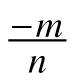2.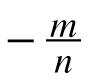3.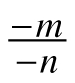4.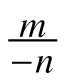5.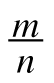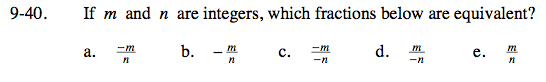Look closely at all the fractions. Which ones look similar?

(a) = (b) = (d),
(c) = (e)